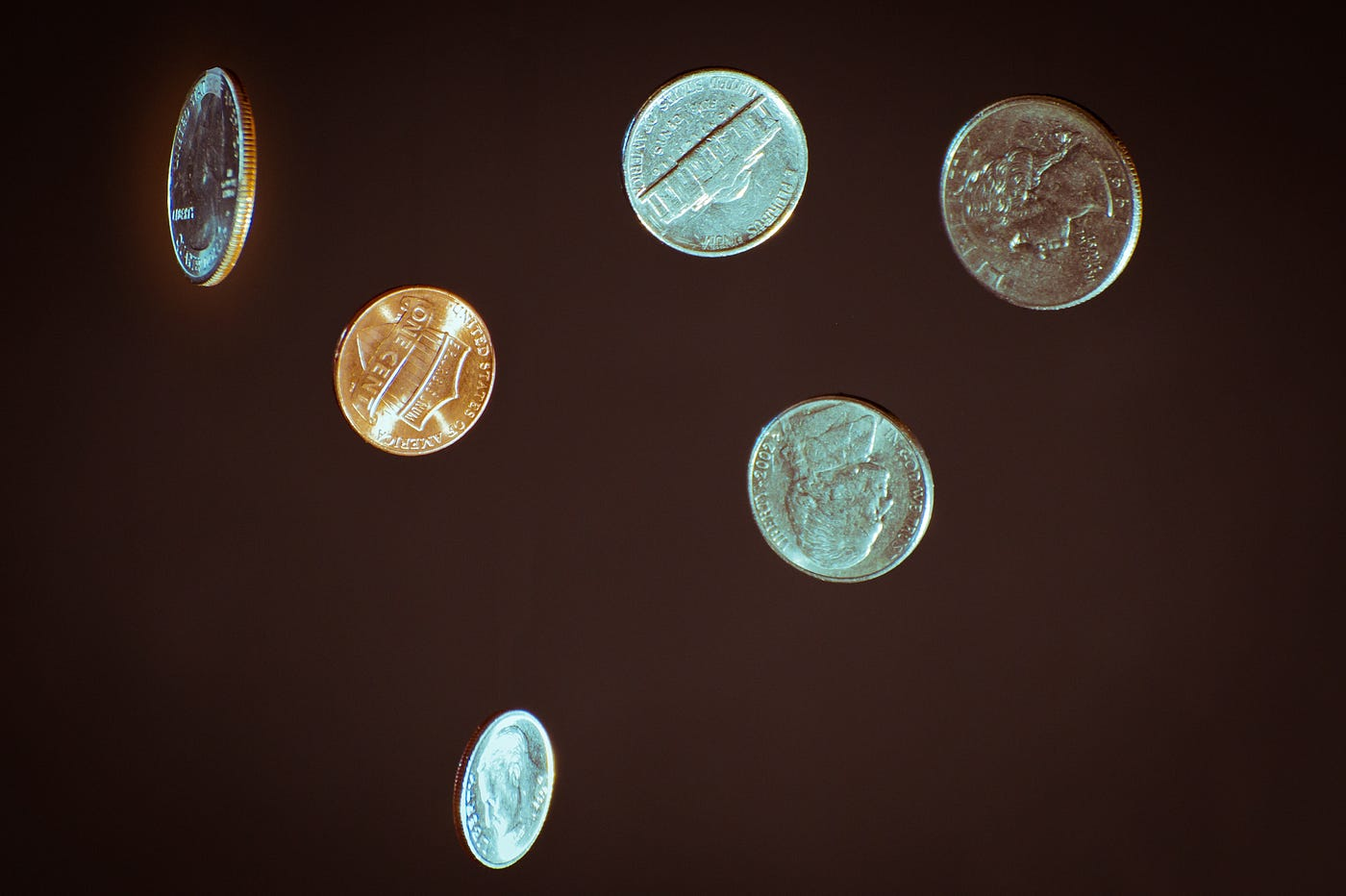# CS50x Cash

## From problem set 1Photo by Chris Briggs on Unsplash

Cash is the easier project we work on the entire course (provided you’re using the CS50 library). It basically asks us to count how many coins we need to use to return a change. Here the cashier wants to use the least amount of coins possible, not mattering how many coins of each are in the cashier’s drawer.

To begin, we open CS50 IDE through the Menu in the course page or by typing ide.cs50.io on your browser. Then procced to create our cash project folder.

`cd ~/pset1mkdir cashcd cashtouch cash.c`

You’ll notice that now we have a folder called cash with a file called cash.c inside. Let’s open it.

`open cash.c`

This is where we’ll start to code! Step by step:

`// Prompt user for a change value   // Make sure it's positive// Round it and store as cents in a variable// Count the least amount of coins needed for the change// Print result`

Reading the Implementation Details in the problem set we learn that we’ll need at least two libraries to make this work: stdio and math. I wanted so much to not use CS50 library, for my own motives (maybe I’ll explain further on), so I added ctype for running manually all the checks cs50 would’ve run for me to make sure the user enters a float. I used scanf(), passed every test, excluding re-prompting after the user entered a char, I could only terminate the program… SO, back to the training wheels, CS50 library included in the header.

So this is what the base of our code should look like:

`#include <stdio.h>#include <math.h>#include <cs50.h>int main(void){ }`

The next step is to prompt the user for a value, his owed change, and make sure that value is valid. For that I’m creating a function. Inside it, I chose a do/while loop for this case, because it makes sure we prompt the user at least one time before moving on, and then re-prompts if condition isn’t met.

Remember that main should always be the first function in the program, by convention, so any other we add need to go after main.

`float getValue(void){    float change;    do    {        change = get_float("Change owed: ");    }    while (change < 0.00);return change;}`

As we now have a function we should remember to add it’s prototype to the header, otherwise the compiler is going to check an error when we call it in main. As I’m also using a custom function to count the coins, let’s add it now.

`float getValue(void);int coins(int cents);`

Moving on, time to call our getValue function in main, store the value it returns and then round our hard earned dollars into cents. Math library has the perfect function to do it! It’s called (surprise) round!

`float owed = getValue();int cents = round(owed * 100);`

From here you can start checking the code to see if it’s working properly. Compile it using make on terminal and run some tests.

`make cash`

An example of intended behavior:

`\$ ./cashChange owed: -0.41Change owed: fooChange owed: 0.41`

We can see that the program keeps asking the user for a valid value until it gets it. What do we do now? Let’s count coins.

`int coins(int cents){    int count = 0;        while (cents > 0)    {        if (cents >= 25)        {            cents -= 25;            count++;        }        else if (cents >= 10)        {            cents -= 10;            count++;        }        else if (cents >= 5)        {            cents -= 5;            count++;        }        else        {            cents -= 1;            count++;        }    }    return count;`

Our coins function takes the rounded cents value we prepared before. Then it uses a loop to check if this value is bigger than each one of our coins, decreasing the value of cents by the value of the coin and adding one to our count every time it’s true. In other words, if the change owed was \$1.16, we transform the number to 116 cents. What the code does is to check if 116 is bigger than 25, if true, decreases it to 91 and add a coin to the counter. A 25 cents coin, but that doesn’t matter for us. It repeats until we have decreased 116 to 16, then it jumps to the next coin value and so on. At the end, we’ll have four 25 cents coins, one ten cents, one five cents, and one one cent. Our count then is 7. That’s what the function will return.

Due to the parameters of the print function required to our last step, we can now finish our code adding one single line to main.

`printf("%d\n", coins(cents));`

Printf is going to print an integer followed by a new line on the terminal, as declared by “%d\n”. What this integer will be is defined by the second parameter, which is our coins function. So the integer is going to be the integer returned by the coins function.

Let’s run the staff checks on the code now.

`~/pset1/cash/ \$ style50 cash.cResults generated by style50 v2.7.4Looks good!~/pset1/cash/ \$ check50 cs50/problems/2020/x/cash...Results for cs50/problems/2020/x/cash generated by check50 v3.1.2:) cash.c exists:) cash.c compiles:) input of 0.41 yields output of 4:) input of 0.01 yields output of 1:) input of 0.15 yields output of 2:) input of 1.6 yields output of 7:) input of 23 yields output of 92:) input of 4.2 yields output of 18:) rejects a negative input like -1:) rejects a non-numeric input of "foo" :) rejects a non-numeric input of ""`

As we can see from the happy faces, everything ok!

Here follows the full code with comments.

--

--